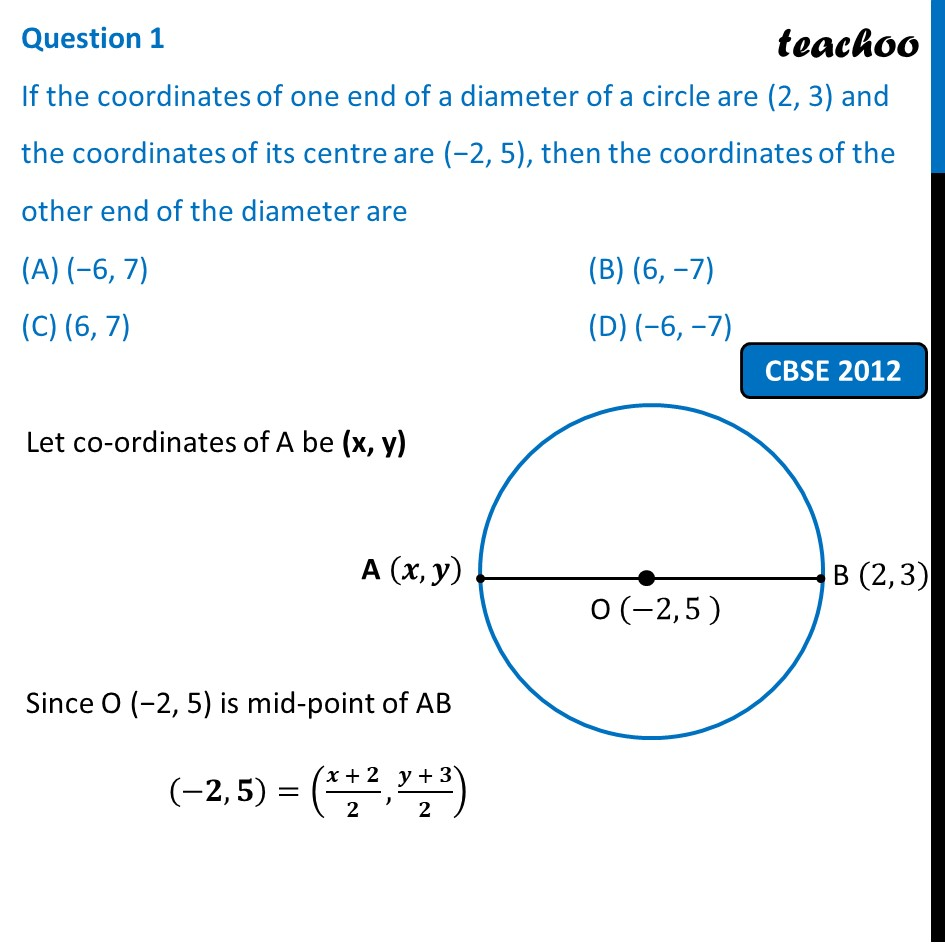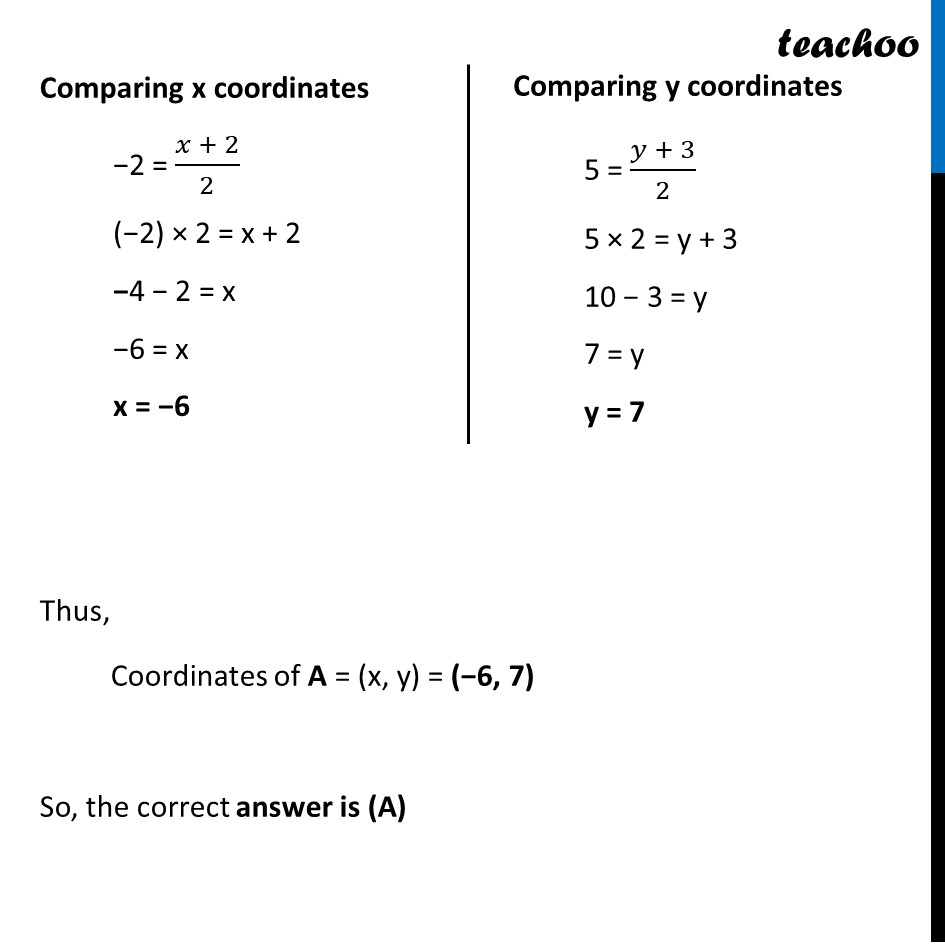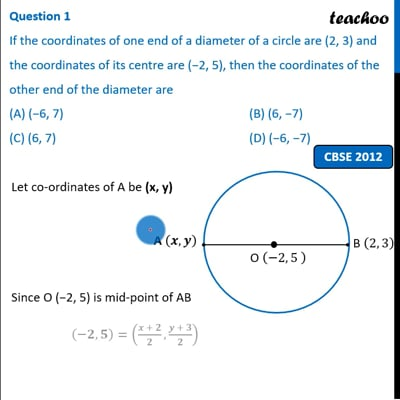Past Year MCQ

Chapter 7 Class 10 Coordinate Geometry
Serial order wise

## (D) (−6, −7)

This question is simlar to Ex 7.2, 7 - Chapter 7 Class 10 Coordinate GeometryThis video is only available for Teachoo black users

Maths Crash Course - Live lectures + all videos + Real time Doubt solving!

### Transcript

Question 1 If the coordinates of one end of a diameter of a circle are (2, 3) and the coordinates of its centre are (−2, 5), then the coordinates of the other end of the diameter are (A) (−6, 7) (B) (6, −7) (C) (6, 7) (D) (−6, −7) Let co-ordinates of A be (x, y) Since O (−2, 5) is mid-point of AB (−𝟐,𝟓)=((𝒙 + 𝟐)/𝟐,(𝒚 + 𝟑)/𝟐) Comparing x coordinates −2 = (𝑥 + 2)/2 (−2) × 2 = x + 2 −4 − 2 = x −6 = x x = −6 Comparing y coordinates 5 = (𝑦 + 3)/2 5 × 2 = y + 3 10 − 3 = y 7 = y y = 7 Thus, Coordinates of A = (x, y) = (−6, 7) So, the correct answer is (A)